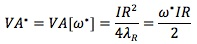### Why should I choose AnalystNotes?

AnalystNotes specializes in helping candidates pass. Period.

The optimal portfolio P* lies at the point where the residual frontier is tangent to the preference line.This implies that the ability of the manager to add value increases as:

• The square of the IR increases. In fact, the key to active management is the information ratio.
• The manager's risk aversion level decreases.

Given our risk aversion level, we all want the investment manager with the highest IR.

#### Practice Question 1

In seeking maximum value added, different investors will differ in:

A. IR: how high the information ratio to take.
B. λ: how aggressive of the investment strategy.
C. ω: how much residual risk to take.

They all seek the manager with the highest IR. A and B will determine the optimal level of residual risk to take.

#### Practice Question 2

The value added by a very good manager (IR = 0.75) with a conservative implementation (λ = 0.15) is:

A. 5%.
B. 1.25%.
C. 0.94%.

0.752/(4 x 0.15) = 0.9375%.

#### Practice Question 3

The key to active management is the:

A. IR: information ratio.
B. λ: risk aversion level.
C. ω: residual risk level.

The value added is determined by IR and λ. The IR has a bigger impact on the value added.

#### Practice Question 4

The value added by a very good manager (IR = 0.75) with an aggressive implementation (λ = 0.05)is:

A. 2.81%.
B. 15%.
C. 3.75%.